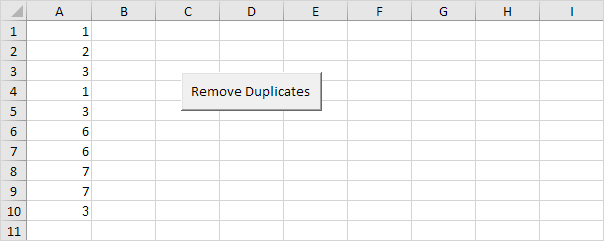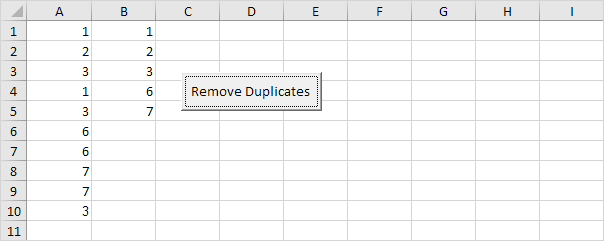# Remove Duplicates

Below we will look at a program in Excel VBA that removes duplicates.

Situation:

In column A we have 10 numbers. We want to remove the duplicates from these numbers and place the unique numbers in column B.1. First, we declare four variables. toAdd of type Boolean, uniqueNumbers of type Integer, i of type Integer, and j of type Integer.

Dim toAdd As Boolean, uniqueNumbers As Integer, i As Integer, j As Integer

2. Next, we write the first number of column A to column B since the first number is always 'unique'.

Cells(1, 2).Value = Cells(1, 1).Value

3. We initialize two variables. We've just added one number to column B, so we initialize uniqueNumbers with the value 1. We set toAdd to True assuming that the next number needs to be added as well (this is not necessarily true of course).

uniqueNumbers = 1

We need to determine whether the second number is 'unique' or not. This can be done in a very easy way. Only if the number is not already in column B, the second number needs to be added to column B.

4. We also need to check this for the third number, fourth number, and so on. We start a For Next loop for this.

For i = 2 To 10

5. Now comes the most important part of the program. If the second number is equal to one of the numbers in column B (so far we only have one unique number), we set toAdd to False because in this case we don't want to add this number! (it is not 'unique'). At the moment uniqueNumbers is still equal to 1, but uniqueNumbers can be a whole list. To check this whole list, we need another For Next loop. Again: if the number we want to add is equal to one of the numbers in this list, toAdd will be set to False and the number will not be added. Add the following code lines:

For j = 1 To uniqueNumbers
If Cells(i, 1).Value = Cells(j, 2).Value Then
End If
Next j

6. Only if toAdd is still True and not set to False, Excel VBA needs to add the number to column B. At the same time, we increment uniqueNumbers by 1 because we have one unique number more now. The following code lines get the job done:

Cells(uniqueNumbers + 1, 2).Value = Cells(i, 1).Value
uniqueNumbers = uniqueNumbers + 1
End If

7. Finally, we set toAdd to True assuming the next number (third number) needs to be added. Again this is not necessarily true.

8. Don't forget to close the loop.

Next i

9. Place your macro in a command button and test it.

Result:Go to Next Chapter: Macro Errors